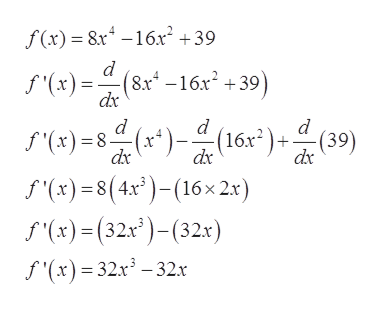# Let f (x) = 8x4 − 16x2 + 39Find the value(s) of c at which f ' changes from positive to negative and list them in increasing order. Enter DNE in any unused answer blanks.c = c = c = Find the values of c at which f ' changes from negative to positive at the following numbers (list in increasing order).c = c = c =

Question
8 views

Let f (x) = 8x4 − 16x2 + 39

Find the value(s) of c at which f ' changes from positive to negative and list them in increasing order.
Enter DNE in any unused answer blanks.

c =
c =
c =

Find the values of c at which f ' changes from negative to positive at the following numbers (list in increasing order).

c =
c =
c =

check_circle

Step 1

Given:

Step 2

Formula used:

Step 3

Differentiate with respect ...help_outlineImage Transcriptionclosef(x) 8x-16x2 +39 d f()(816 +39) f(x)=8 (16)(39) f (x)=8(4x)-(16x 2x) f(x)(32x)-(32x) (8x -16x2 +39) dx d d dx dx f'(x) 32x3-32x fullscreen

### Want to see the full answer?

See Solution

#### Want to see this answer and more?

Solutions are written by subject experts who are available 24/7. Questions are typically answered within 1 hour.*

See Solution
*Response times may vary by subject and question.
Tagged in